# 和屌丝一起学SWIFT-自动引用计数(ARC)

[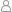孤狼 |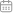2016-04-28 11:21:45 |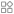Swift |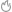2114°C |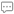0条评论 ]本教程仅用于初学SWIFT同学使用，内容由本人（孤狼）学习过程中笔记编写，本教程使用SWIFT版本为2.0。本教程内容可以自由转载，但必须同时附带本声明，或注明出处。PAEA.CN版权所有。SWIFT中会使用自动引用计数(ARC)来跟踪管理应用内存,当检测到一个类的实例不会再被使用的时候,就会自动去释放这个实例所占用的内存.一般情况这个过程是自动的,不需要人为参与,不过,总有不起作用的时候.所以学习他还是很有意义的.

[工作机制]

``class A{    init(){        print("实例创建了");    }    deinit{        print("实例销毁");    }}//为了能够销毁实例,我们依然使用一个可选类型来创建实例var a1:A?;var a2:A?;var a3:A?;//开始创建实例a1=A();     //输出"实例创建了"//接下来,我们把这个实例引用出去,因为都是一个实例,所以这里不会再次调用A的构造器.a2=a1;a3=a2;//接着我们开始销毁a2=nil;     //我们销毁了a2的引用,不过没有任何反应,因为实例的强引用还有a1和a3//我们继续销毁a1a1=nil;     //这个时候,我们的强引用还剩a3,不过只要用一个引用都不会销毁哦.//我们全部销毁看看.a3=nil;     //输出"实例销毁"``

[循环强引用]

``class B{    var bb:C?;    init(){        print("B创建了");    }    deinit{        print("B销毁了");    }}class C{    var cc:B?;    init(){        print("C创建了");    }    deinit{        print("C销毁了");    }}var b:B?=B();     //输出"B创建了"var c:C?=C();     //输出"C创建了"//接着,我们因为业务需要他们之间互相引用.b?.bb=c;c?.cc=b;//用完之后我们销毁b和cb=nil;          //无输出c=nil;          //无输出``

[弱引用]

``class B{    weak var bb:C?;    init(){        print("B创建了");    }    deinit{        print("B销毁了");    }}class C{    weak var cc:B?;     //这里我们使用弱引用.    init(){        print("C创建了");    }    deinit{        print("C销毁了");    }}var b:B?=B();     //输出"B创建了"var c:C?=C();     //输出"C创建了"//接着,我们因为业务需要他们之间互相引用.b?.bb=c;c?.cc=b;//用完之后我们销毁b和cb=nil;          //检测到c对b的引用只是弱引用,b不存在强引用了,所以可以销毁b,输出"B销毁了",//同时检测到弱引用cc的存在,所以cc被变为nilc=nil;          //检测到b已经被销毁,bb引用已经不存在,所以不存在强引用,可以销毁c,输出"C销毁了"``

TIP:弱引用情况下,如果一个引用被销毁,那么ARC会赋值nil来表示不存在,所以引用必须是变量类型.同时我们也要注意弱引用的使用,因为是弱引用,一旦对象没有强引用则会被释放,可能会出现意想不到的提前释放问题.

[无主引用]

``class B{    var bb:C?;    init(){        print("B创建了");    }    deinit{        print("B销毁了");    }}class C{    unowned var cc:B;     //这里我们使用无主引用.    init(x:B){        print("C创建了");        cc=x;    }    deinit{        print("C销毁了");    }}var b:B?=B();       //输出"B创建了"b?.bb=C(x:b!);      //输出"C创建了" 同时cc无主引用到b//用完之后我们销毁bb=nil;              //输出"B销毁了,C销毁了"//b没有强引用,开始销毁.销毁后,因为c失去了强引用而自动销毁.``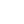赞赏一个鸡腿